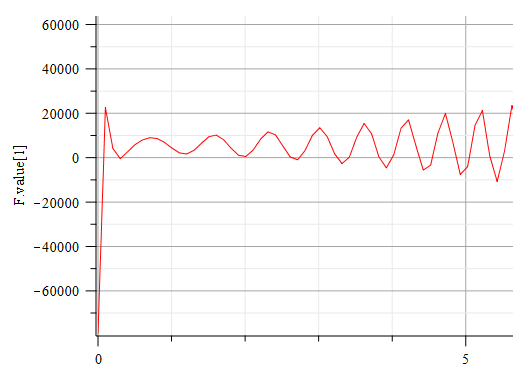## Find the focus of an ellipse with a tangential gen...

Etude d'un cas particulier a := 5: b := 7: k := 9: A := [a, 0]: B := [0, b]: #A et B fixes P := [t, 0]: Q := [0, k/t]:#P et Q 2 points mobiles cir := -a*x-b*y+x^2+y^2 = 0: sol := solve(subs(y = 5, cir), x): cen := [solve(diff(cir, x)), solve(diff(cir, y))]: x0 := sol: y0 := 5: M := [x0, y0]: R := sqrt(cen^2+cen^2): beta := arctan(diff(solve(EQ(M, cen), y), x)): Recherche des valeurs de t pour que les 2 droites soient perpendiculaires eq := t^2*(y0-b)+t*(a*b-a*y0+b*x0-k)-x0*(a*b-k) = 0; sol := solve(eq, t); t := sol; tp := sol; P1 := [t, 0]; Q1 := [0, k/t]; PQ1 := simplify(x*(-a*b+b*t+k)+y*t*(t-a)-t*(-a*b+b*t+k)) = 0:#1ere tangente PQ2 := simplify(x*(-a*b+b*tp+k)+y*tp*(tp-a)-tp*(-a*b+b*tp+k)) = 0:#2ième tangente P2 := [tp, 0]; Q2 := [0, k/tp]; CIR := implicitplot(cir, x = -4 .. 8, y = -4 .. 12, color = red); Fig := proc (alpha) local Dr1, DR1, Dr2, DR2, N, u0, v0, Po, t, tp, sol; global a, b, k, cen, R; u0 := cen+R*cos(alpha); v0 := cen+R*sin(alpha); N := [u0, v0]; sol := solve(t^2*(v0-b)+t*(b*u0-a*v0+a*b-k)-u0*(a*b-k) = 0, t); t := sol; tp := sol; Dr1 := simplify(x*(-a*b+b*t+k)+y*t*(t-a)-t*(-a*b+b*t+k)) = 0; DR1 := implicitplot(Dr1, x = -4 .. 8, y = -4 .. 12, color = brown); Dr2 := simplify(x*(-a*b+b*tp+k)+y*tp*(tp-a)-tp*(-a*b+b*tp+k)) = 0; DR2 := implicitplot(Dr2, x = -4 .. 8, y = -4 .. 12, color = pink); Po := pointplot([N[]], symbol = solidcircle, color = [black], symbolsize = 8); display([Po, DR1, DR2]) end proc; DrPQ1 := implicitplot(PQ1, x = -4 .. 22, y = -4 .. 12, color = blue); DrPQ2 := implicitplot(PQ2, x = -4 .. 22, y = -4 .. 12, color = blue); Points := pointplot([A[], B[], M[], P1[], P2[], Q1[], Q2[], cen[]], symbol = solidcircle, color = [green], symbolsize = 10); T := plots:-textplot([[A[], "A"], [B[], "B"], [M[], "M"], [P1[], "P1"], [P2[], "P2"], [Q1[], "Q1"], [Q2[], "Q2"], [cen[], "cen"]], font = [times, 10], align = {below, left}); n := 19; display([seq(Fig(2*i*Pi/n), i = 0 .. n), Fig(beta), CIR, DrPQ1, DrPQ2, Points, T], scaling = constrained, size = [500, 500]); I would find out the focus of the ellipse. Thank you.

## Homotopy Analysis Method, NOPH package and solutio...

Hello, dear forum users!

Does anyone use the method of homotopy analysis (HAM) and the NOPH package in their work. (moderator: link)It seems to me that only HAM can help.

There are no developments, as I do not own the NOPH package.

## Error in Battery operated 4 wheeled driven electri...

Hi all,

I am new to maplesim and trying to learn it.

While simulation of the battery operated 4 wheeled driven electric vehicle

it tells can't find solution.

Same time if i connect a fixed reference all four wheels are running (same place) but not moving since the frame fixed.

HELP ME TO SOLVE THIS.

thank you..

## How to make  "with(combinat)"work in Maple Linux...

When I type "with(combinat)" in Maple Linux, I got the following erros message:

"Error, invalid input: with expects its 1st argument, pname, to be of type {`module`, package}, but received combinat"

So how to make it work?

Many thanks.

## `Anew Technique of intial Boundary Value Problem U...

Hello! hope you all be fine. i m running a code for `Anew Technique of intial Boundary Value Problem Using ADM `(Elaf*Jaafar*Ali)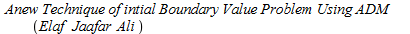but my code is for positive integer and i want to run it for fractional can anyone help me please code file is attached

## How to simply a equation like textbook, Maple use ...

The pdf shows the derivation procedure of Image from 1.2.1 to 1.2.5. However, when we obtain the (7) in pdf, which is the

expansion of 1.2.4, we hope to see the hamiltonian operator in the output.  We obtain the (10) in pdf (Maple). Actually, it is the expansion of 1.2.5. However, it is too cockamamie compared with that (1.2.5) in the textbook.

Hence, I hope to show the hamiltonian operator in the (10) in PDF. I hope to see the simplest type of equation.

How could I do? Appreciate the kind help from anyone!

The Maple code is also uploaded.(1)(2)(3)(4)(5)(6)(7)(8)(9)(10)20200619.pdf## Compiler package setup...

Error, (in Compiler:-Setup) unable to write to initialization file, C:\Program Files\Maple 2020\bin.X86_64_WINDOWS/launch.ini, possibly due to insufficient permissions
NULL;

when choose bat file

but i see sufficient permission

any w should i s

choose a particular compiler or even visual studio i have in my system

where can i find if anything else

## Error importing a big Excel data file xlsx...

Hello,

I try import a big Excel file with the first row as column labels for performing statistical etc.

I use this command :

data := Import(FileTools:-JoinPath(["d:", "directory", "subset2.xlsx"], platform = "windows"))

i need a dataframe for performing statistical with column label as first row, but i have this error :

Error, (in Import) invalid input: MatrixToDataFrame uses a 3rd argument, columns, which is missing

I can not understand what is this error...so, what i can do ?

Thanks, regards

## wrong result working with differential forms?...

This is my notebook

 >>>M >M >(1)
 M >(2)
 M >
 >I don't understand why computing the wedge product of two differential forms gives me an output which prints the product of both. Why  don't it just return d rho ^ dt?

## How to plot a function at a point 0....

Dear Maple Users @acer @Carl Love @Kitonum @Preben Alsholm ,

Greetings.

How to plot a function "Am" for various values of "kt"(eg: kt=-1..1) at a point x=0.

Am vs kt.

The code has provided below.

ktplot.mw

## implicitplot3d+contourplot...

Hi experts

with(plots):
#epsilon:=5*Pi/2:
gammaa:=0.4:
gammao:=0.1:
gammab:=0.002:
omegab:=100:
X:=60:
Omega:=6*Pi:
g:=10:
sigmag:=5*gammaa:
a:=1/(sigmag*sqrt(2*Pi)):

#deltao:=1:
D7:=evalf(a*int(g*Omega*(gammao/2-I*Delta)*exp(-0.5*((Delta-deltao)/sigmag)^2)/(gammao^2/4+Delta^2),Delta=-infinity..infinity)):
D8:=evalf(0.5*a*int(g^2*gammao*exp(-0.5*((Delta-deltao)/sigmag)^2)/(gammao^2/4+Delta^2), Delta=-infinity..infinity)):
D9:=evalf(a*int(g^2*Delta*exp(-0.5*((Delta-deltao)/sigmag)^2)/(gammao^2/4+Delta^2), Delta=-infinity..infinity)):
b1 := (deltao-2*X^2*omegab*Y/(gammab^2+omegab^2)-D9)^2:

f:=Y*((gammaa+D8)^2+(b1))=Im(D7)^2+(epsilon-Re(D7))^2:
#P1:=implicitplot(f,deltao=-70..50,Y=0..1,numpoints=100,axes=boxed,thickness=2,color=black,font=[1,1,20],tickmarks=[4, 3],linestyle=1);

implicitplot3d(f,Y = 0 .. 1,deltao=-40..40,epsilon=0..0.4,numpoints=100,labels=[Y,deltao,E],tickmarks=[3,3,3], style = surface, color = "Niagara Azure");

The above code to plot the implicitplot3d ,

first the figures are not smooth

second thing is how to make contourplot at epsilon= 5*Pi/2.

## Colouring inequalities...

Hello everyone,

I would have a question about how to colour a region of complicated inequalities.

I'm curious about the region where my 'lambdas' are all negatives regarding the parameters 'pm2' and 'am2', but I always find difficulties even if I try with implicitplot or inequal commands. Also, I would like to put into the same graph with different colours when they are separately smaller than 0.

I've already tried plenty of things, I hopefully attached some pics by snipping tool about them.  And here is an example of my problem:

`p61w:= implicitplot([lambda61 <= 0], pm2 = 0 .. 1, am2 = 0 .. 1)`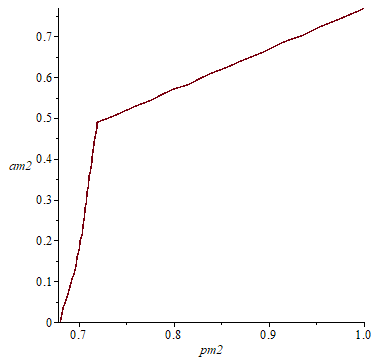`in61 := inequal(lambda61 <= 0, pm2 = 0 .. 1, am2 = 0 .. 1)`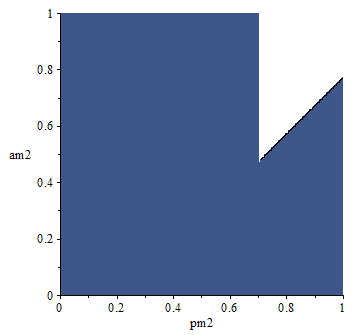`display(in61,p61w)`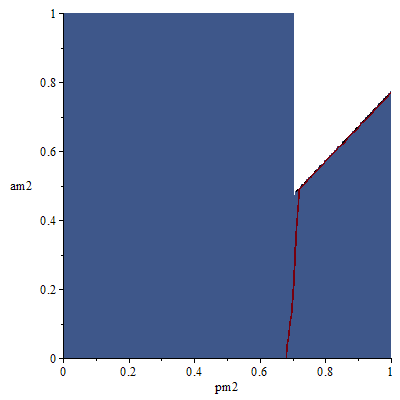So, the main issue that it is not just coloured where my condition is true, but elsewhere too. In plot 'in61' as you can see, there is a region approx. pm2=0..0.6 where it shouldn't be coloured since my condition is not true there. In the graph; 'display(in61, p61w)',  it is visible that under the red line should be just coloured however my 'inequal' command coloured it outside too.

Also, I tried with implicitplot, for example:

`p6 := implicitplot([lambda61 <= 0], pm2 = 0 .. 1, am2 = 0 .. 1, filled = true, coloring = [blue, white], transparency = .5)`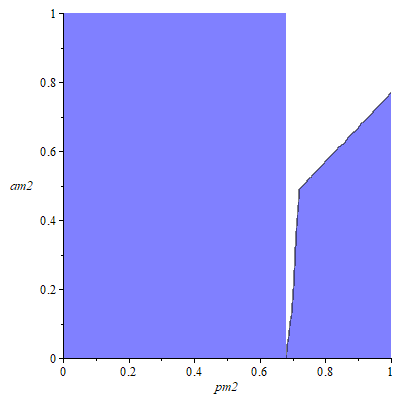But the result is similarly wrong. And I'm not sure why it happens and how can I fix it.

(It's okay if for example for these particular lambdas (lambda61,lambda62) never negatives together, but I have more systems and I don't think it works properly)

I read similar questions too but I couldn't find an answer so, I would very appreciate any suggestions.

This is my first post in Maple, so please let me know if I have mistaken something.

## How to produce implicit plot within loop...

Here is my try:

with(plots):
epsilon:=5*Pi/2:
gammaa:=0.4:
gammao:=0.1:
gammab:=0.002:
omegab:=100:
X:=60:
Omega:=6*Pi:
g:=10:
sigmag:=5*gammaa:
a:=1/(sigmag*sqrt(2*Pi)):
for deltao from 0 to 4 do
D7:=evalf(a*int(g*Omega*(gammao/2-I*Delta)*exp(-0.5*((Delta-deltao)/sigmag)^2)/(gammao^2/4+Delta^2),Delta=-infinity..infinity)):
D8:=evalf(0.5*a*int(g^2*gammao*exp(-0.5*((Delta-deltao)/sigmag)^2)/(gammao^2/4+Delta^2), Delta=-infinity..infinity)):
D9:=evalf(a*int(g^2*Delta*exp(-0.5*((Delta-deltao)/sigmag)^2)/(gammao^2/4+Delta^2), Delta=-infinity..infinity)):
b1:=deltao-(2*X^2*omegab*Y^2)/(omegab^2+gammab^2)-D9:
f:=epsilon-D7=Y*(gammaa+D8+I*(b1)):
P1:=implicitplot(f,Y^2=0..10,delta0=0..1,numpoints=1000,axes=boxed,thickness=2,color=black,font=[1,1,20],tickmarks=[4, 3],linestyle=1);
end do:

display(P1);

The function (f)  is an implicit function of Y^2

so my code above is an example to plot Y^2 against deltao

note that Y is complex

## How to measure the contact force?...

Hello guys,

im not an expert of maplesim, I'm working on one of the default quarter car models (first pic).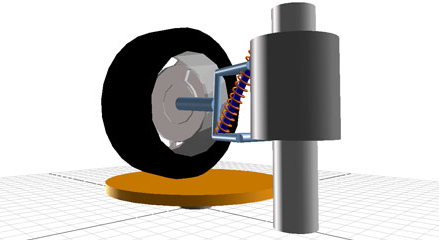I set a probe to measure the instant contact force between the tire and the post, but the graph shows that the force reaches both posite and negative values (as if the post were pulling back down the tire, is that right?)

What l have to do in order to see the real contact force graph? i just want to see the real positive value or 0.